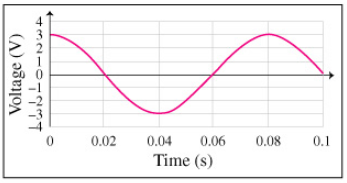# Problem: Part AWhat is the maximum voltage Vmax of the source?Part BWhat is the average voltage Vavg of the source?Part CWhat is the root-mean-square voltage Vrms of the source? Express your answer to three significant figures.Part DWhat is the period T of the source? Express your answer in seconds to two significant figures.Part EWhat is the frequency f of the source? Express your answer in hertz to three significant figures.Part FWhat is the angular frequency, f, of the source? Express your answer in radians per second to three significant figures.

###### FREE Expert Solution

$\overline{)\begin{array}{rcl}{\mathbf{V}}_{\mathbf{rms}}& {\mathbf{=}}& \frac{{\mathbf{V}}_{\mathbf{max}}}{\sqrt{\mathbf{2}}}\end{array}}$

$\overline{)\begin{array}{rcl}{\mathbf{f}}& {\mathbf{=}}& \frac{\mathbf{1}}{\mathbf{T}}\end{array}}$

$\overline{)\begin{array}{rcl}{\mathbf{\omega }}& {\mathbf{=}}& \mathbf{2}\mathbf{\pi f}\end{array}}$

Part A

Vmax corresponds to maximum amplitude of the wave.###### Problem DetailsPart A

What is the maximum voltage Vmax of the source?

Part B

What is the average voltage Vavg of the source?

Part C

What is the root-mean-square voltage Vrms of the source? Express your answer to three significant figures.

Part D

What is the period T of the source? Express your answer in seconds to two significant figures.

Part E

What is the frequency f of the source? Express your answer in hertz to three significant figures.

Part F

What is the angular frequency, f, of the source? Express your answer in radians per second to three significant figures.